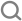maths

A .
B .
C .
D .
physics

A .
B .
C .
D .
physics

# The given diagram shows four processes i.e., isochoric, isobaric, isothermal  and adiabatic. The correct assignment of the process, in the same order is given by :-A .
d a c b
B .
a d c b
C .
a d b c
D .
d a b c
physics

A .
B .
C .
D .
chemistry

biology

history

biology

physics